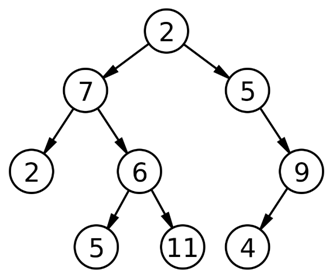Q:

# Leftmost and Rightmost Nodes of Binary Tree

Given a Binary Tree, Print the corner nodes at each level. The node at the leftmost and the node at the rightmost.

Example:For this tree output will be: 2 7 5 2 9 5 4

Solution:

Of course the solution includes level order traversal.

Algorithm:

FUNCTION printCorner (root): prints corner nodes (leftmost, rightmost) of the tree

Prerequisite: Queue q, input binary tree root

```FUNCTION printCorner(Node *root)
1.  Declare a queue to store pointer to tree nodesq;
2.  Declare store=0 which will print the rightmost node;
3.  Declare temp to store DeQueued element from queue ;
4.  Declare count=0 which will help to print the leftmost node;
5.  Print the root->data first
6.  IF (root->left)
EnQueue (q,root->left);
IF (root->right)
EnQueue (q,root->right);
EnQueue (q, NULL); //to indicate end of first level (root only)
7.  While(q is not empty){
temp=DeQueue(q);
Increment count;
//temp is not NULL && temp is pointer to the first node (leftmost)
IF(temp && count==1)
Print temp->data;
ELSE IF(temp) //for other nodes rather than the leftmost one
store=temp->data; //update store each time with latest node value
END IF-ELSE
IF (temp==NULL) //end of previous level
IF (q is not empty)
EnQueue(q,NULL);
END IF
count=0; //re-define countas 0 for next level
Print store; //store consist of rightmost of last level node value
ELSE
IF(temp->left is not NULL)
EnQueue(q, temp->left);
IF(temp->right is not NULL)
EnQueue(q, temp->right);
END IF-ELSE
END WHILE
END FUNCTION
```

Example with explanation:N.B: The nodes are represented by their respective values.

For the above example:

Nodes are represented with their respective values for better understanding.

```In the main we call printCorner (2)
----------------------------------------------------

printCorner (2)
store=0
count=0
Print root//2
root->left is not NULL
q.push(root->left)//7
root->right is not NULL
q.push(root->right)//5
q.push(NULL)
----------------------------------------------------

1st iteration:
q is not empty
temp= q.front()=7
q.pop()
Queue status:
5 NULL
Count=1
Temp not NULL and count is 1
Print 7
7->left not NULL
q.push (7->left) //2
7->right not NULL
q.push(7->right) //6
Queue status:
5 NULL 2 6
----------------------------------------------------

2nd iteration:
q is not empty
temp= q.front()=5
q.pop()
count=2; //incremented
Queue status:
2 6
temp not NULL and count is not 1
Update store with temp->data// store=5
5->leftNULL
5->right not NULL
q.push(5->right) //9
Queue status:
NULL 2 6 9
----------------------------------------------------

3rd iteration:
q is not empty
temp= q.front()=NULL
q.pop()
count=3 //incremented
Queue status:
2 6 9
Temp is NULL
Print store // 5
Count re-initialized to 0
q is not empty
q.push(NULL)
Queue status:
2 6 9 NULL
----------------------------------------------------

4th iteration:
q is not empty
temp= q.front()=2
q.pop()
count=1; //incremented
temp is not NULL && count is 1
Print temp//2
Queue status:
6 9 NULL
2->left NULL
2->right NULL

Queue status:
6 9 NULL
----------------------------------------------------

5th iteration:
q is not empty
temp= q.front()=6
q.pop()
count=2; //incremented
temp is not NULL , but count not 1
store=6
Queue status:
9 NULL
6->left  not NULL
q.push(6->left) //5
6->right not NULL
q.push(6->left) //11
Queue status:
9 NULL 5 11
----------------------------------------------------

6th iteration:
q is not empty
temp= q.front()=9
q.pop()
count=3; //incremented
temp is not NULL , but count not 1
store=9
Queue status:
NULL 5 11
9->left  not NULL
q.push(9->left) //4
9->right NULL
Queue status:
NULL 5 11 4
----------------------------------------------------

7th iteration:
q is not empty
temp= q.front()=NULL
q.pop()
Queue status:
5 11 4
Count=4//incremented
Temp is NULL
Print store // 9
Count re-initialized to 0
q is not empty
q.push(NULL)
Queue status:
5 11 4 NULL
----------------------------------------------------

8th iteration:
q is not empty
temp= q.front()=5
q.pop()
Queue status:
11 4 NULL
Count=1; //incemented
Temp is not NULL and count is 1
Print temp->data //5
5->left   NULL
5->right NULL

Queue status:
11 4 NULL
----------------------------------------------------

9th iteration:
q is not empty
temp= q.front()=11
q.pop()
Queue status:
4 NULL
Count=2; //incemented
temp is not NULL and count is not 1
store= temp->data //11
11->left   NULL
11->right NULL

Queue status:
4 NULL
----------------------------------------------------

10th iteration:
q is not empty
temp= q.front()=4
q.pop()
Queue status:
NULL
Count=4; //incemented
temp is not NULL and count is not 1
store= temp->data //4
4->left   NULL
4->right NULL
----------------------------------------------------

11th iteration:
q is not empty
temp= q.front()=NULL
q.pop()
Queue status:
Empty
Count=5//incremented
Temp is NULL
Print store // 5
Count re-initialized to 0
qis empty
Queue status:
Empty
----------------------------------------------------
Iteration ends

Queue status:
Empty
Output:
2 7 5 2 9 5 4```

C++ implementation:

``````#include <bits/stdc++.h>
using namespace std;

// tree node is defined
class Node{
public:
int data;
Node *left;
Node *right;
};

void printCorner(Node *root){
queue<Node*> q;
int store=0;
Node* temp;
int count=0;

cout<<root->data<<"\n";
if(root->left)
q.push(root->left);
if(root->right)
q.push(root->right);
q.push(NULL);
while(!q.empty()){
temp=q.front();
q.pop();
count++;
if(temp && count==1)
cout<<temp->data<<" ";
else if(temp)
store=temp->data;
if(temp==NULL){
count=0;
cout<<store<<"\n";
if(!q.empty()){
q.push(NULL);
}
}
else{
if(temp->left)
q.push(temp->left);
if(temp->right)
q.push(temp->right);
}
}
}

// creating new node
Node* newnode(int data)
{
Node* node = (Node*)malloc(sizeof(Node));
node->data = data;
node->left = NULL;
node->right = NULL;
return(node);
}

int main() {
//**same tree is builted as shown in example**
cout<<"same tree is builted as shown in example\n";
Node *root=newnode(2);

root->left= newnode(7);
root->right= newnode(5);
root->right->right=newnode(9);
root->right->right->left=newnode(4);
root->left->left=newnode(2);
root->left->right=newnode(6);
root->left->right->left=newnode(5);
root->left->right->right=newnode(11);

cout<<"Printing the right most & left most nodes of binary tree...:"<<endl;
printCorner(root);

return 0;
}
``````

Output

```same tree is builted as shown in example
Printing the right most & left most nodes of binary tree...:
2
7 5
2 9
5 4```